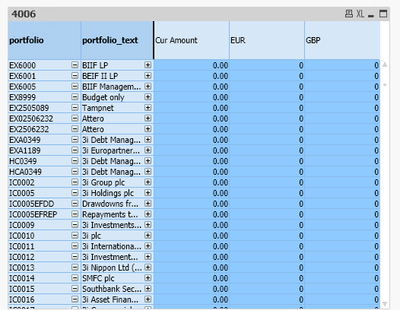# QlikView App Dev

Discussion Board for collaboration related to QlikView App Development.

cancel
Showing results for
Did you mean:Creator III

## How to restrict values in a dimension

Hello

I have table of portfolio values.  I only want to show the values that begin with IC (some begin with EX)

I'm not sure if I do this as a calculated dimension or within the expression.

I tried the below in the expresson so I suspect mayabe i need to do this as a calculated dimension but not sure how

sum({\$ <period = {">=\$(vStartPeriod_CY)<=\$(vEndPeriod_CY)"}, groupcoa ={4006}, portfolio = {'IC*'} >} cur_amount )

Many thanks

PaulLabels (2)

• ### QLIKVIEW Script

1 Solution

Accepted SolutionsCreator

Hi @paul_ripley ,

it is good to create a new field in script editor and use that field in the table. please try replacing single quotes with double quotes in the set expression for portfolio. try using the below expression.

sum({\$ <period = {">=\$(vStartPeriod_CY)<=\$(vEndPeriod_CY)"}, groupcoa ={4006}, portfolio = {"IC*"} >} cur_amount )

2 RepliesCreator

Hi @paul_ripley ,

it is good to create a new field in script editor and use that field in the table. please try replacing single quotes with double quotes in the set expression for portfolio. try using the below expression.

sum({\$ <period = {">=\$(vStartPeriod_CY)<=\$(vEndPeriod_CY)"}, groupcoa ={4006}, portfolio = {"IC*"} >} cur_amount )Creator III
Author

Hi

Just FYI  I created a calculated dimension as so  =if(wildmatch(portfolio, 'IC*'), portfolio)

Kind regards

Paul